Question

There is a monopoly producer of smartphones facing the following demand Q = 400 - 2P (where Q is # of smartphones). Its cost is given by C = 100Q.

(a) What are the equilibrium quantity and price of smartphones sold by the monopolist?

(b) If the government imposes an ad valorem tax of 20% on smartphones, what happens to the equilibrium quantity and price? What is the tax revenue collected? What is the tax incidence of this tax? (HINT: assume that the tax reduces MR not increases MC).

(c) If instead of the ad valorem tax of 20% the government wants to impose an equivalent excise (per unit) tax on smartphones, what tax amount should it use? What tax revenue would be collected? What would be the tax incidence? Why is the tax incidence on the monopolist higher in (b)? Please explain. (HINT: use the difference between the MR and price in your explanation).

d) If the government imposes an excise tax of \$42 on smartphones instead, what happens to the equilibrium quantity and price and tax revenue? What is the tax incidence now? How does the tax incidence here compare to (c)? Please explain why this is the case. (e) If the ad valorem tax rate goes up above 20%, what would you expect to happen with the tax incidence and why? Please explain.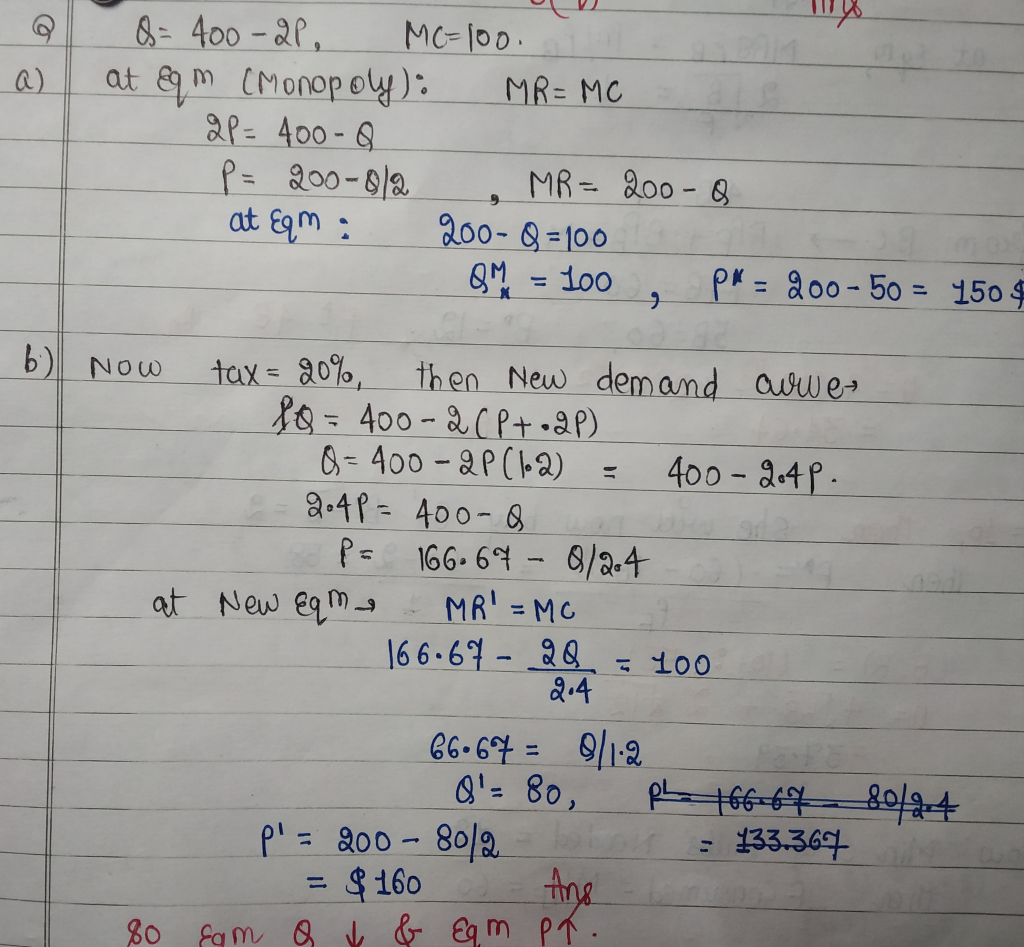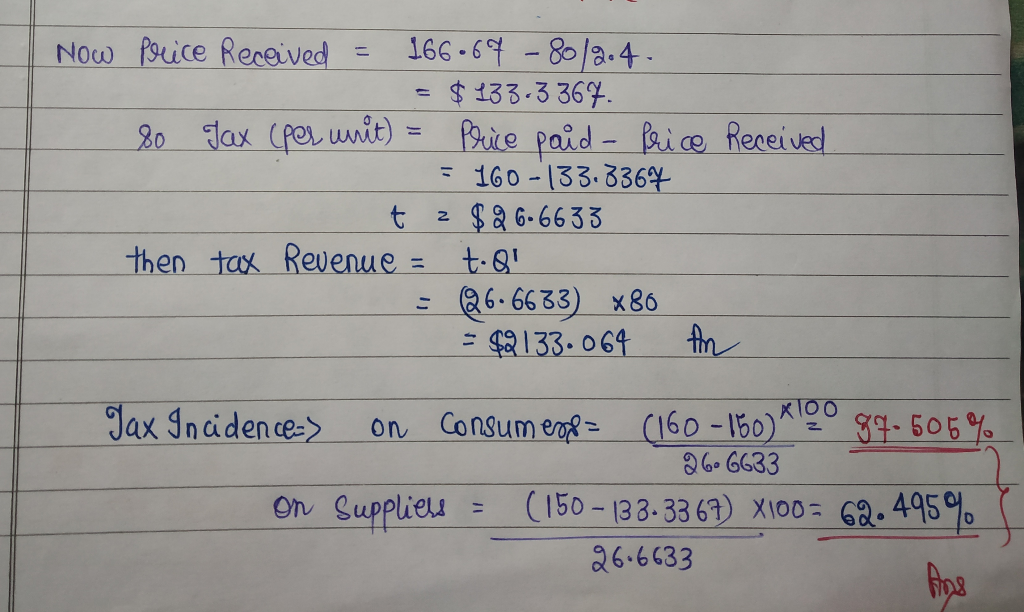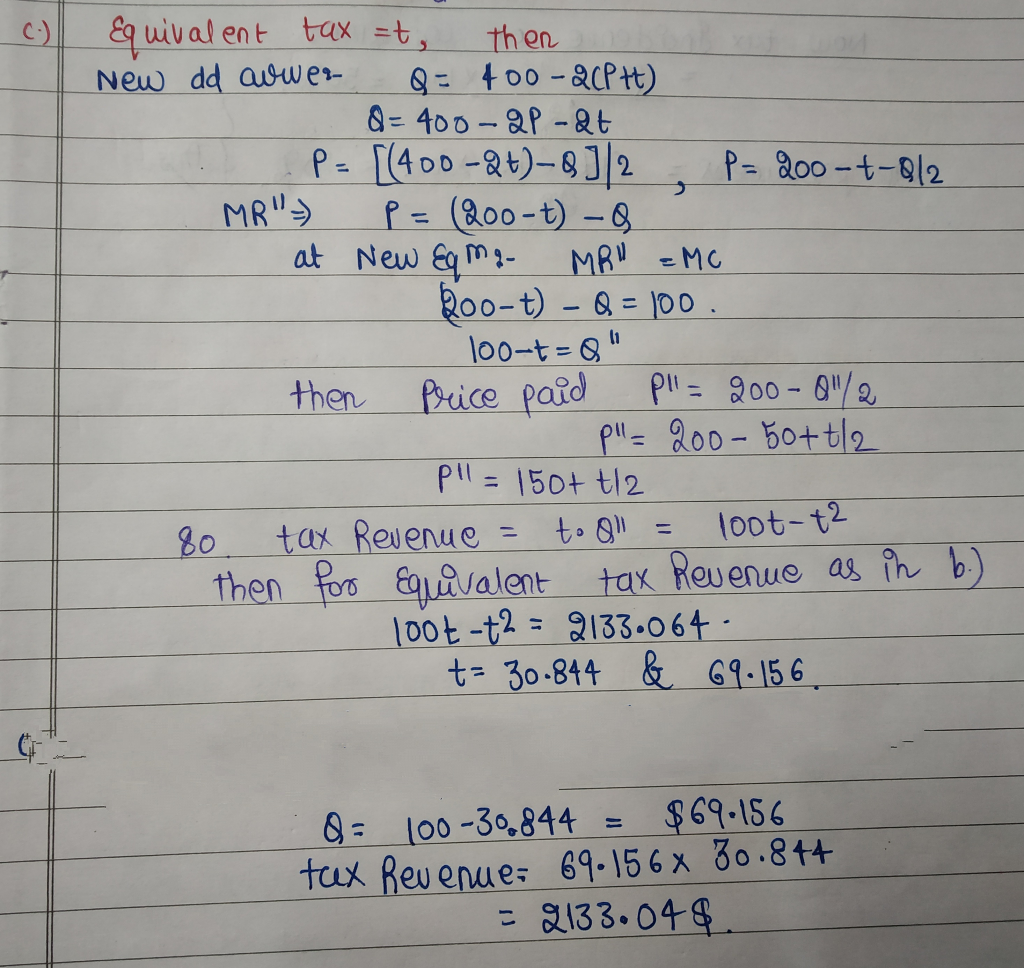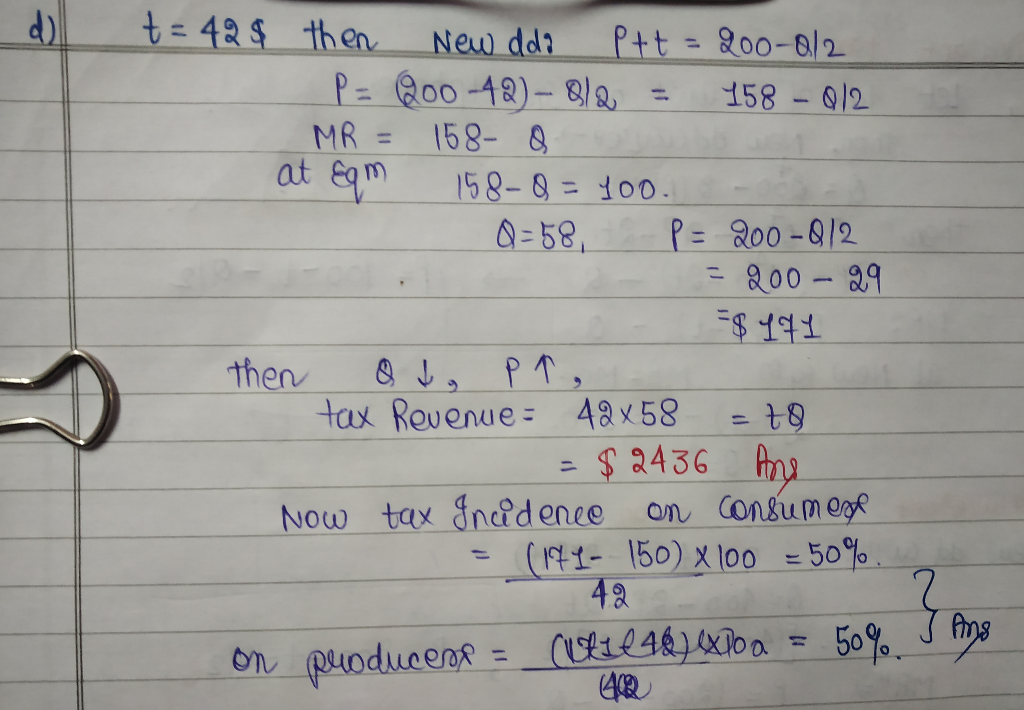#### Earn Coins

Coins can be redeemed for fabulous gifts.

Similar Homework Help Questions
• ### 5. Consider a market supply and demand represented by the following: Q. = 3P - 60...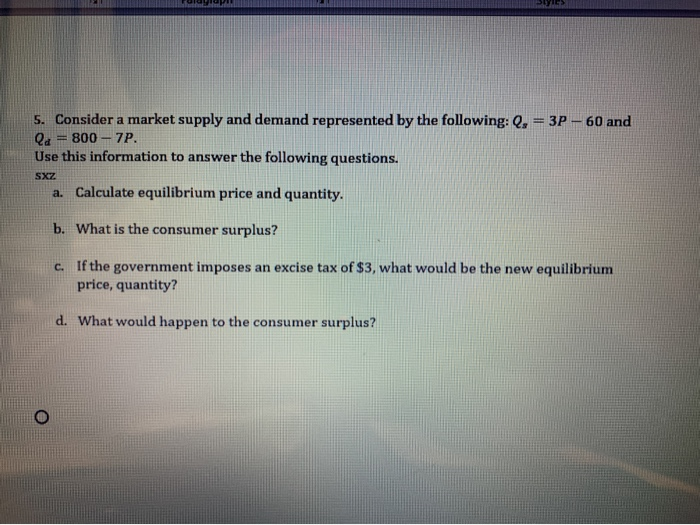5. Consider a market supply and demand represented by the following: Q. = 3P - 60 and la = 800 - 7P. Use this information to answer the following questions. SXZ a. Calculate equilibrium price and quantity. b. What is the consumer surplus? c. If the government imposes an excise tax of \$3, what would be the new equilibrium price, quantity? d. What would happen to the consumer surplus?

• ### Questions 2-4 examine the market for Zeds. The demand for Zeds is given by Q- 12,500-500P...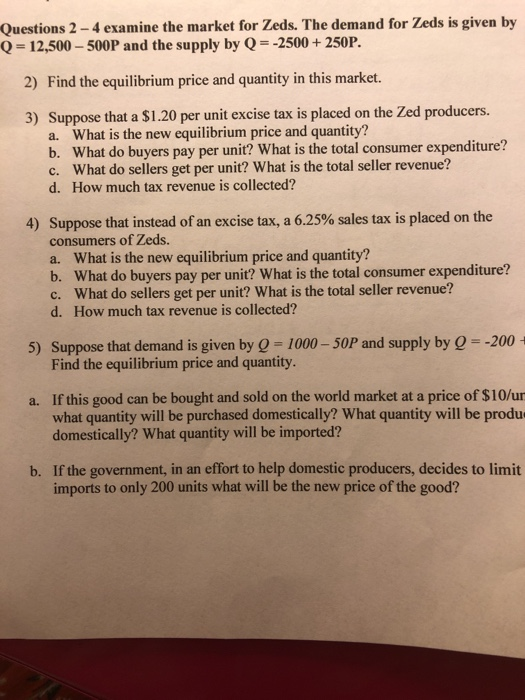Questions 2-4 examine the market for Zeds. The demand for Zeds is given by Q- 12,500-500P and the supply by Q 2500+250P. 2) Find the equilibrium price and quantity in this market. 3) Suppose that a S1.20 per unit excise tax is placed on the Zed producers. What is the new equilibrium price and quantity? b. a. What do buyers pay per unit? What is the total consumer expenditure? What do sellers get per unit? What is the total seller...

• ### Question 2: Consider the market for ice cream where the demand is given by QD 20-...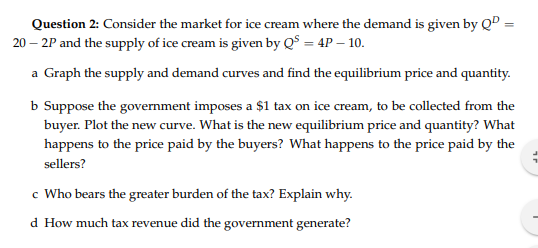Question 2: Consider the market for ice cream where the demand is given by QD 20- 2P and the supply of ice cream is given by QS 4P 10 a Graph the supply and demand curves and find the equilibrium price and quantity b Suppose the government imposes a \$1 tax on ice cream, to be collected from the buyer. Plot the new curve. What is the new equilibrium price and quantity? What happens to the price paid by the...

• ### 3. The demand and supply for wine are given by Q-20-P and Q-3P, respectively. P is...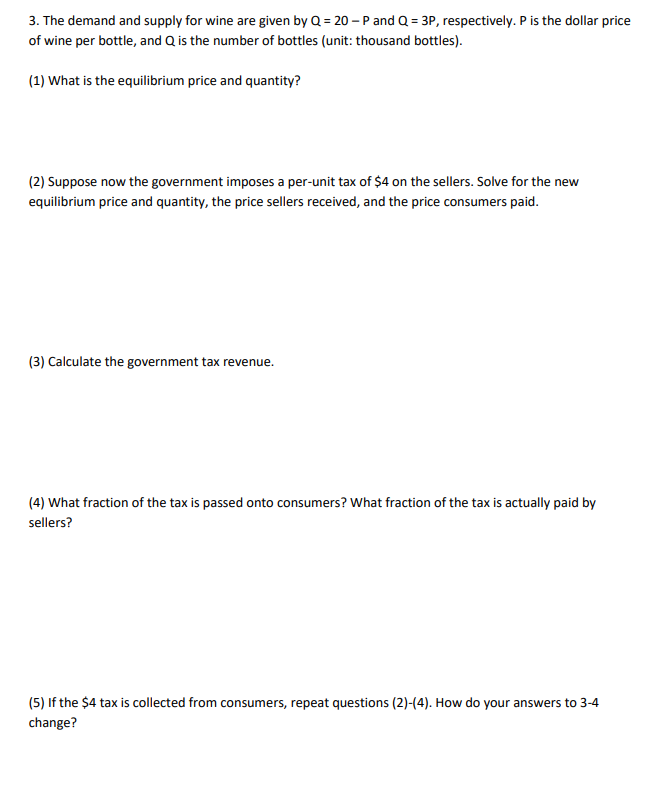3. The demand and supply for wine are given by Q-20-P and Q-3P, respectively. P is the dollar price of wine per bottle, and Q is the number of bottles (unit: thousand bottles). (1) What is the equilibrium price and quantity? (2) Suppose now the government imposes a per-unit tax of \$4 on the sellers. Solve for the nevw equilibrium price and quantity, the price sellers received, and the price consumers paid. (3) Calculate the government tax revenue. (4) What...

• ### Suppose the supply of a good is given by the equation Q 800P 2,400, and the...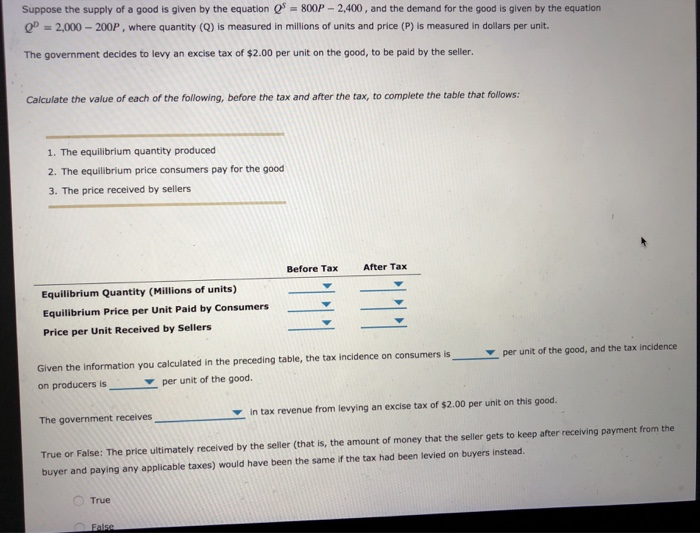Suppose the supply of a good is given by the equation Q 800P 2,400, and the demand for the good is given by the equation 2,000-200P , where quantity (Q) is measured in millions of units and price (P) is measured in dollars per unit. The government decides to levy an excise tax of \$2.00 per unit on the good, to be paid by the seller. Calculate the value of each of the following, before the tax and after the...

• ### Consumer & Producer Surplus If QP = 450 - P and Q* = 2P - 150:...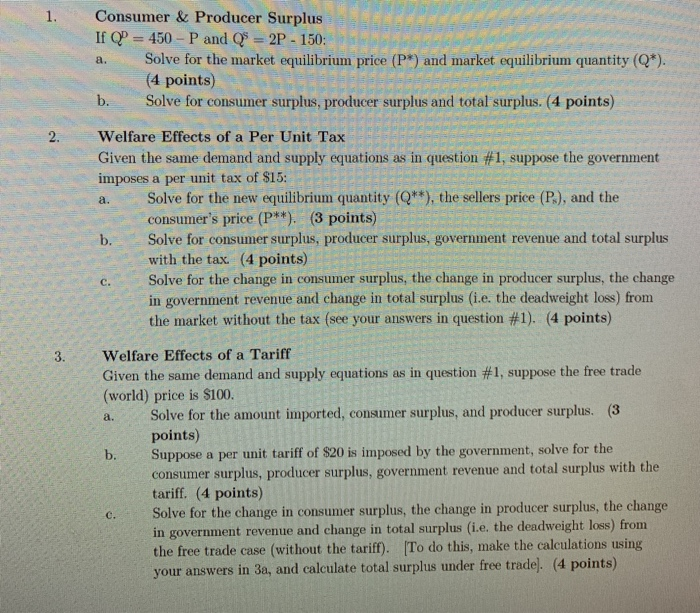Consumer & Producer Surplus If QP = 450 - P and Q* = 2P - 150: a. Solve for the market equilibrium price (P) and market equilibrium quantity (Q*). (4 points) b. Solve for consumer surplus, producer surplus and total surplus. (4 points) 2. Welfare Effects of a Per Unit Tax Given the same demand and supply equations as in question #1, suppose the government imposes a per unit tax of \$15: 22 a. Solve for the new equilibrium quantity...

• ### The government imposes a 20% ad valorem tax on a monopoly with cost curve C(Q) =...

The government imposes a 20% ad valorem tax on a monopoly with cost curve C(Q) = 10 + 4 Q^2 facing demand curve 200-5Q. Round your answers to one significant digit after the decimal point if needed. What is Consumer surplus What is Producer surplus What are Government revenues What is the Deadweight loss

• ### A monopolist faces the following demand curve: Q = 260-2P Where Q is the weekly production...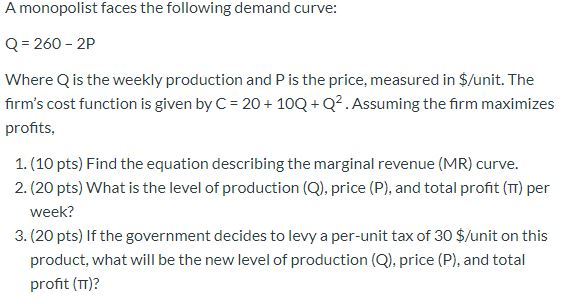A monopolist faces the following demand curve: Q = 260-2P Where Q is the weekly production and P is the price, measured in \$/unit. The firm's cost function is given by C= 20 + 10Q+Q2. Assuming the firm maximizes profits, 1. (10 pts) Find the equation describing the marginal revenue (MR) curve. 2. (20 pts) What is the level of production (Q), price (P), and total profit (TT) per week? 3. (20 pts) If the government decides to levy a...

• ### Suppose demand in a market is P 120 Q 240 2P This is a monopoly market,...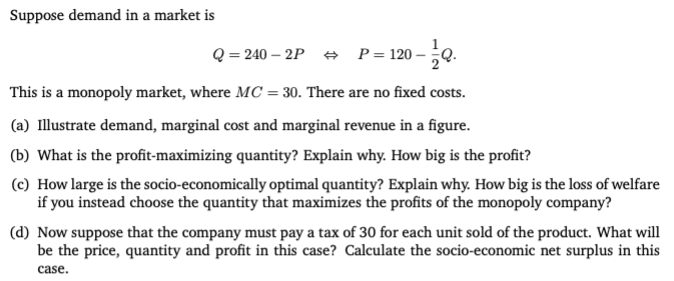Suppose demand in a market is P 120 Q 240 2P This is a monopoly market, where MC = 30. There are no fixed costs. (a) Illustrate demand, marginal cost and marginal revenue in a figure (b) What is the profit-maximizing quantity? Explain why. How big is the profit? (e) How large is the socio-economically optimal quantity? Explain why. How big is the loss of welfare if you instead choose the quantity that maximizes the profits of the monopoly company?...

• ### 3. The market illustrated below has inverse demand p(Q) = 130 - 3Q and industry-wide marginal...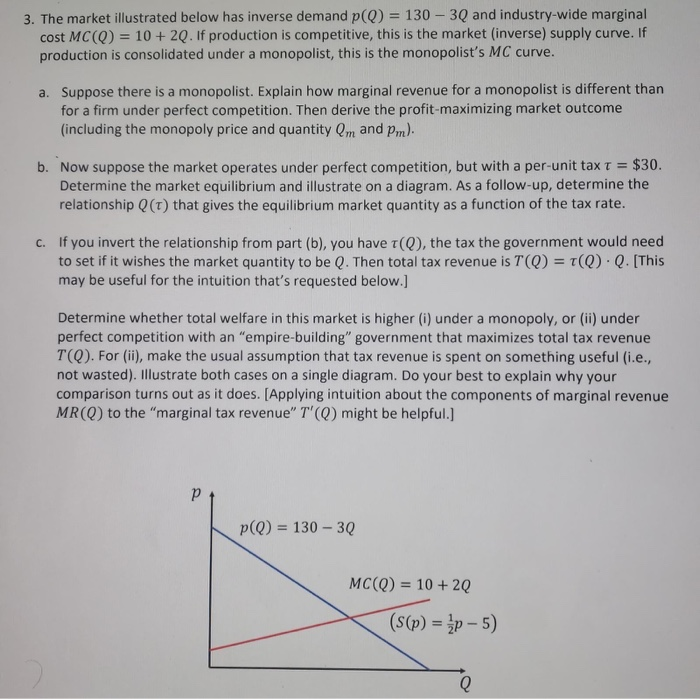3. The market illustrated below has inverse demand p(Q) = 130 - 3Q and industry-wide marginal cost MCQ) = 10 + 2Q. If production is competitive, this is the market (inverse) supply curve. If production is consolidated under a monopolist, this is the monopolist's MC curve. a. Suppose there is a monopolist. Explain how marginal revenue for a monopolist is different than for a firm under perfect competition. Then derive the profit-maximizing market outcome (including the monopoly price and quantity...